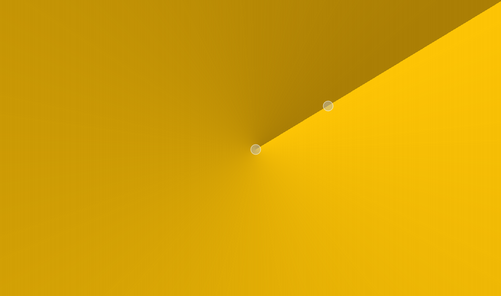The QConicalGradient class is used in combination with QBrush to specify a conical gradient brush. More...

 Header: #include CMake: find_package(Qt6 COMPONENTS Gui REQUIRED) target_link_libraries(mytarget PRIVATE Qt6::Gui) qmake: QT += gui Inherits: QGradient

## Public Functions

 QConicalGradient(qreal cx, qreal cy, qreal angle) QConicalGradient(const QPointF ¢er, qreal angle) QConicalGradient() qreal angle() const QPointF center() const void setAngle(qreal angle) void setCenter(const QPointF ¢er) void setCenter(qreal x, qreal y)

## Detailed Description

Conical gradients interpolate interpolate colors counter-clockwise around a center point.The colors in a gradient is defined using stop points of the QGradientStop type, i.e. a position and a color. Use the QGradient::setColorAt() or the QGradient::setStops() function to define the stop points. It is the gradient's complete set of stop points that describes how the gradient area should be filled. If no stop points have been specified, a gradient of black at 0 to white at 1 is used.

In addition to the functions inherited from QGradient, the QConicalGradient class provides the angle() and center() functions returning the start angle and center of the gradient.

Note that the setSpread() function has no effect for conical gradients. The reason is that the conical gradient is closed by definition, i.e. the conical gradient fills the entire circle from 0 - 360 degrees, while the boundary of a radial or a linear gradient can be specified through its radius or final stop points, respectively.

## Member Function Documentation

Constructs a conical gradient with the given center (cx, cy), starting the interpolation at the given angle. The angle must be specified in degrees between 0 and 360.

Constructs a conical gradient with the given center, starting the interpolation at the given angle. The angle must be specified in degrees between 0 and 360.

Constructs a conical with center at (0, 0) starting the interpolation at angle 0.

### qreal QConicalGradient::angle() const

Returns the start angle of the conical gradient in logical coordinates.

### QPointF QConicalGradient::center() const

Returns the center of the conical gradient in logical coordinates.

Sets angle to be the start angle for this conical gradient in logical coordinates.

### void QConicalGradient::setCenter(const QPointF &center)

Sets the center of this conical gradient in logical coordinates to center.